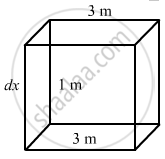Department of Pre-University Education, KarnatakaPUC Karnataka Science Class 11
Advertisement Remove all ads

# Water is Filled in a Rectangular Tank of Size 3 M × 2 M × 1 M. (A) Find the Total Force Exerted by the Water on the Bottom Surface on the Tank. (B) Consider a Vertical Side of Area 2 M × 1 M. - Physics

Sum

Water is filled in a rectangular tank of size 3 m × 2 m × 1 m. (a) Find the total force exerted by the water on the bottom surface on the tank. (b) Consider a vertical side of area 2 m × 1 m. Take a horizontal strip of width δx metre in this side, situated at a depth of x metre from the surface of water. Find the force by the water on this strip. (c) Find the torque of the force calculate in part (b) about the bottom edge of this side.
(d) Find the total force by the water on this side.
(e) Find the total torque by the water on the side about the bottom edge. Neglect the atmospheric pressure and take g = 10 ms−2

Advertisement Remove all ads

#### Solution

Dimensions of the rectangular tank:
Length, l = 3 m
Breadth, b = 2 m
Height, h = 1 m
Area of the bottom surface of the tank, A = 2 × 3 = 6 m

Density of water, ρw = 1000 kgm−3(a) Total force exerted by water on the bottom surface of the tank:

$\text{ f = Ah}\rho_\text{w g}$

$= 6 \times 1 \times {10}^3 \times 10$

$= 6 \times {10}^4 = 60, 000 \text{N}$

(b) Force exerted by water on the strip of width δx:

$\text{ df = p } \times \text{ A } = \left( \text{ x}\rho_\text{w g} \right) \times A$

$= \text{x} \times {10}^3 \times 10 \times 2 \times \delta \text{x}$

$= 20, 000\text{x}\delta \text{x N}$

(c) Inside the tank, the water force acts in every direction due to adhesion. Therefore, torque is given by

$\text{di} = F \times r$

$= 20, 000 \times \delta x(1 - x) N$

(d) Total force exerted by water on the side about the bottom edge (F) :

$\text{F} = \int_0^1 20, 000 \text{ x}\delta\text{ x}$

$\Rightarrow F = 20, 000 \left[ \frac{\text{x}^2}{2} \right]_0^1$

$= 10, 000 \text{N}$

(e) Torque by the water on the side (T):

$\tau = 20, 000 \times \int_0^1 \text{x}\delta \text{x}\left( 1 - x \right)$

$= 20, 000 \left[ \frac{\text{x}^2}{2} - \frac{\text{x}^3}{3} \right]_0^1$

$= 20, 000 \times \left[ \frac{1}{2} - \frac{1}{3} \right]$

$= \frac{20, 000}{6} \text{ Nm }= \frac{10000}{3} \text{ Nm }$

Is there an error in this question or solution?
Advertisement Remove all ads

#### APPEARS IN

HC Verma Class 11, 12 Concepts of Physics 1
Chapter 13 Fluid Mechanics
Q 8 | Page 274
Advertisement Remove all ads
Advertisement Remove all ads
Share
Notifications

View all notifications

Forgot password?